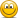VaR versus standard deviation

annaleevance2000

New Member
Subscriber
Hello @David Harper CFA FRM , sorry to bother you again, but I did not know where to put this question about Meissner's Example 1.2 of VaR for a two-asset portfolio. (Should I create a new thread for questions like this in the future?)

On the table, the 'Deviate' is written as 2.326. However, if my sleep-deprived brain is not wrong, shouldn't the 1-tailed (for VaR) 99% confidence interval critical value be 2.576? Or is this 'Deviate' the long square-root equation called the 'standard deviation of a two-asset portfolio' as listed on page 7 of the study notes?

Thank you for taking the time to answer my simple questionsDavid Harper CFA FRM

David Harper CFA FRM
Staff member
Subscriber
Hi @annaleevance2000 I don't want to take the time to pull up the references (it's time consuming for me to attempt reconciliations in the content) especially given the basic nature of your question.

Re: shouldn't the 1-tailed (for VaR) 99% confidence interval critical value be 2.576?
No, if the distribution is the standard normal, the 99% VaR equals NORM.S.INV(0.99) = 2.326. Always true, full stop. It is unnecessary to write "the one-tailed 99% VaR" because VaR is always one-tailed. In fact, I would not write that b/c it suggest incorrectly that two-tailed VaR might be an option. VaR needs (i) a distributional assumption, (ii) a horizon, and a confidence level. It does not need a "one tailed" instruction.

Standard deviation is (two-sided, if you will) dispersion measure. It has the familiar form, appearing in this forum hundreds of times; e.g., https://en.wikipedia.org/wiki/Standard_deviation

So maybe a historical stock price series exhibits a volatility of 10.0% per annum. That means "standard deviation of returns of 10.0% annualized." It's two-sided in the sense it's including both directions (squaring the deviations). If the distribution were somehow normal, the associated 99.0% VaR would be 10% * 2.326 = 23.3%. In this way, we scaled (multiplied) the volatility to identify the one-sided quantile (aka, deviate) of this normal distribution. VaR is not volatility. Parametric (analytical) VaR might scale standard deviation, because that's convenient. I hope that's helpful.

Last edited:

annaleevance2000

New Member
Subscriber
Hello David,
Thank you for your response. I appreciate it very much.

Anna